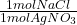## Silver chloride is virtually insoluble in water so that the reaction appears to go to completion. How many grams of solid NaCl must be added

Question

Silver chloride is virtually insoluble in water so that the reaction appears to go to completion. How many grams of solid NaCl must be added to 25.0 mL of 0.366 M AgNO3 solution to completely precipitate the silver

in progress 0
5 months 2021-08-10T05:29:46+00:00 1 Answers 68 views 0

## Answers ( )

0.535 g

Explanation:

The reaction that takes place is:

• NaCl + AgNO₃ → AgCl + NaNO₃

First we calculate how many AgNO₃ moles are there in 25.0 mL of a 0.366 M solution, using the definition of molarity:

• Molarity = moles / liters
• moles = Molarity * liters

Converting 25.0 mL to L ⇒ 25.0 / 1000 = 0.025 L

• moles = 0.366 M * 0.025 L = 0.00915 mol AgNO₃

Then we convert AgNO₃ moles into NaCl moles:

• 0.00915 mol AgNO₃ *= 0.00915 mol NaCl

Finally we convert NaCl moles into grams, using its molar mass:

• 0.00915 mol NaCl * 58.44 g/mol = 0.535 g